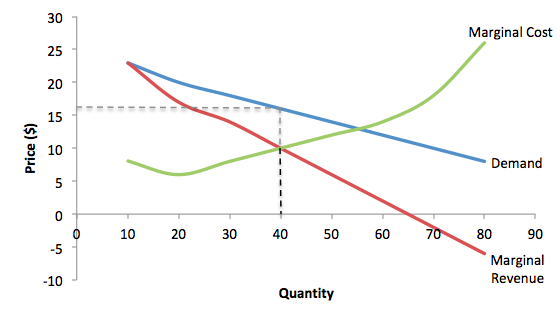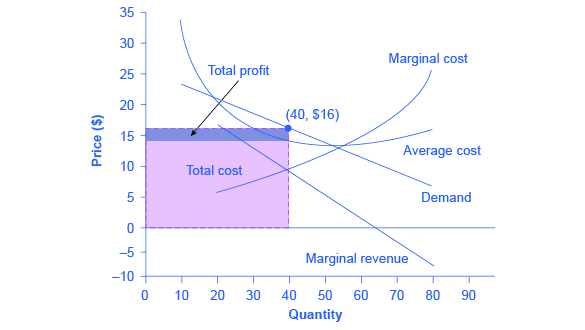## Profit Maximization under Monopolistic Competition

### Learning Objectives

• Describe how a monopolistic competitor chooses price and quantity using marginal revenue and marginal cost
• Graph and interpret a monopolistically competitive firm’s average, marginal, and total cost curves
• Compute total revenue, profits, and losses for monopolistic competitors using the demand and average cost curves

## Choosing the Profit-Maximizing Output and Price

The monopolistically competitive firm decides on its profit-maximizing quantity and price in much the same way as a monopolist. A monopolistic competitor, like a monopolist, faces a downward-sloping demand curve, and so it will choose some combination of price and quantity along its perceived demand curve.

As an example of a profit-maximizing monopolistic competitor, consider the Authentic Chinese Pizza store, which serves pizza with cheese, sweet and sour sauce, and your choice of vegetables and meats. Although Authentic Chinese Pizza must compete against other pizza businesses and restaurants, it has a differentiated product. The firm’s perceived demand curve is downward sloping, as shown in Figure 1 and the first two columns of Table 1.Figure 1. How a Monopolistic Competitor Chooses its Profit Maximizing Output and Price. To maximize profits, the Authentic Chinese Pizza shop would choose a quantity where marginal revenue equals marginal cost, or Q where MR = MC. Here it would choose a quantity of 40 and a price of $16. Table 1. Revenue and Cost Schedule Quantity Price Total Revenue Marginal Revenue Total Cost Marginal Cost 10$23 $230$340
20 $20$400 $17$400 $6 30$18 $540$14 $480$8
40 $16$640 $10$580 $10 50$14 $700$6 $700$12
60 $12$720 $2$840 $14 70$10 $700 –$2 $1,020$18
80 $8$640 –$6$1,280 $26 The combinations of price and quantity at each point on the demand curve can be multiplied to calculate the total revenue that the firm would receive, which is shown in the third column of Table 1. The fourth column, marginal revenue, is calculated as the change in total revenue divided by the change in quantity. The final columns of Table 1 show total cost, marginal cost, and average cost. As always, marginal cost is calculated by dividing the change in total cost by the change in quantity, while average cost is calculated by dividing total cost by quantity. The following example shows how these firms calculate how much of its product to supply at what price. ### How a Monopolistic Competitor Determines How Much to Produce and at What Price The process by which a monopolistic competitor chooses its profit-maximizing quantity and price resembles closely how a monopoly makes these decisions process. First, the firm selects the profit-maximizing quantity to produce. Then the firm decides what price to charge for that quantity. Step 1. The monopolistic competitor determines its profit-maximizing level of output. In this case, the Authentic Chinese Pizza company will determine the profit-maximizing quantity to produce by considering its marginal revenues and marginal costs. Two scenarios are possible: • If the firm is producing at a quantity of output where marginal revenue exceeds marginal cost, then the firm should keep expanding production, because each marginal unit is adding to profit by bringing in more revenue than its cost. In this way, the firm will produce up to the quantity where MR = MC. • If the firm is producing at a quantity where marginal costs exceed marginal revenue, then each marginal unit is costing more than the revenue it brings in, and the firm will increase its profits by reducing the quantity of output until MR = MC. In this example, MR and MC intersect at a quantity of 40, which is the profit-maximizing level of output for the firm. Step 2. The monopolistic competitor decides what price to charge. When the firm has determined its profit-maximizing quantity of output, it can then look to its perceived demand curve to find out what it can charge for that quantity of output. On the graph, this process can be shown as a vertical line reaching up through the profit-maximizing quantity until it hits the firm’s perceived demand curve. For Authentic Chinese Pizza, it should charge a price of$16 per pizza for a quantity of 40.

Although the process by which a monopolistic competitor makes decisions about quantity and price is similar to the way in which a monopolist makes such decisions, two differences are worth remembering. First, although both a monopolist and a monopolistic competitor face downward-sloping demand curves, the monopolist’s perceived demand curve is the market demand curve, while the perceived demand curve for a monopolistic competitor is based on the extent of its product differentiation and how many competitors it faces. Second, a monopolist is surrounded by barriers to entry and need not fear entry, but a monopolistic competitor who earns profits must expect the entry of firms with similar, but differentiated, products.

## Calculating Profits

Once the monopollstic competitor has determined the profit-maximizing quantity of output to supply, the next step is to calculate how much profit it is earning. We use the same process that was used with perfect competition and monopoly. This is illustrated in Figure 2, using the data from Table 2, which extends the data from to Table 1 to include average total cost in the last column.Figure 2. Computing Profit for a Monopolistic Competitor. To calculate profit, start from the profit-maximizing quantity, which is 40. Next find total revenue which is the area of the rectangle with the height of P = $16 times the base of Q = 40. Next find total cost which is the area of the rectangle with the height of AC =$14.50 times the base of Q = 40. The difference between the two areas is profit, the small rectangle above total cost in the figure.

Table 2. Revenue and Cost Schedule, including Average Cost
Quantity Price Total Revenue Marginal Revenue Total Cost Marginal Cost Average Cost
10 $23$230 $340$34
20 $20$400 $17$400 $6$20
30 $18$540 $14$480 $8$16
40 $16$640 $10$580 $10$14.50
50 $14$700 $6$700 $12$14
60 $12$720 $2$840 $14$14
70 $10$700 –$2$1,020 $18$14.57
80 $8$640 –$6$1,280 $26$16

We start by identifying the profit-maximizing level of output, where marginal revenue equals marginal cost. This is Q = 40. Next, look for the profit margin, the difference between price and average cost. The price is $16, which you can read off the demand curve for quantity equals 40. The average cost is$14.50, which you can read off the average cost curve for quantity equals 40. The profit margin is $16.00 –$14.50 = $1.50 for each unit that the firm sells. Total profit is the profit margin times the quantity or$1.50 x 40 = $60. Alternatively, we can compute profit as total revenue minus total cost. Total revenue is price times quantity or$16.00 x 40 = $640. This is the area of the rectangle that starts at the origin, goes up to a price of$16, goes over to the demand curve, down to the quantity of 40 and back to the origin. Total cost is average cost times quantity or $14.50 x 40 =$580. This is the area of the rectangle that starts at the origin, goes up the vertical axis to an average cost of $14.50, goes over to the average cost curve, down to the quantity of 40 and back to the origin. Profit is the difference between the two areas,$640 – $580 =$60. This is shown graphically as the area of the rectangle on top of total cost, or the price minus average cost, times quantity. Note that if the firm was earning zero economic profits, the rectangles of total revenue and total cost would be the samethere would be no profit rectangle. The break-even point occurs where the demand curve intersects with average cost, so P = AC. Note also that if the firm was making a loss, the negative profit (i.e. loss) would be the rectangle on top of total revenue.

## Contribute!

Did you have an idea for improving this content? We’d love your input.